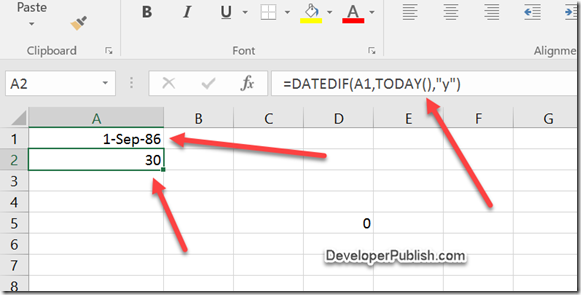# How to Calculate Age in Microsoft Excel 2016 ?

One of the simplest ways to calculate the age of a person in Microsoft Excel 2016 by providing the date of birth as input is using the DATEDIF formula.

# How to Calculate Age in Microsoft Excel 2016 ?

Assume that the Cell A1 contains the date of birth of the person and you need to calculate the age and display it in the cell A2 , your formula would like this

=DATEDIF(A1,TODAY(),”y”)Alternatively , you can also use NOW() instead of TODAY() in the formula.

=DATEDIF(A1,NOW(),”y”)